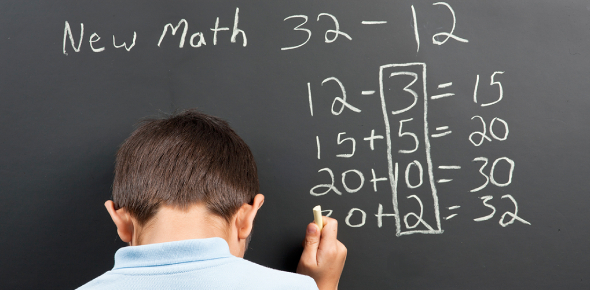# 5th Grade Math Quiz: Test!

10 Questions | Attempts: 1915Settings.

• 1.
Two bases lie on the upper and lower surfaces of a cylinder.
• A.

True

• B.

False

• 2.
The hands of a clock at 3.00 shows acute angle.
• A.

True

• B.

False

• 3.
Thirty four thousand two hundred and eighty four = 34, 284
• A.

True

• B.

False

• 4.
Write the number name of 4,00,000 = ?
• 5.
1, 3, 6, ___, 15, ____, 28
• 6.
____  is a common to number among square and triangular numbers.
• 7.
5 km 700 m + 12 km 450 m = _____________
• A.

19 km 150m

• B.

18 km150 m

• C.

7 km 350 m

• 8.
75 m 15 cm x 5  = ______
• A.

375 m 75 cm

• B.

300 m 75 cm

• C.

80 m 20 cm

• 9.
55 km 75 cm - 23 km 40 cm  =  _______________
• A.

30 km 35 m

• B.

79 km 15 m

• C.

32 km 35m

• 10.
Ram works on his computer from morning 10 'o' clock to evening 3.30. How long does he work on his computer?
• A.

13.30 hours

• B.

6.30 hours

• C.

5.30 hours

## Related TopicsBack to top
×

Wait!
Here's an interesting quiz for you.